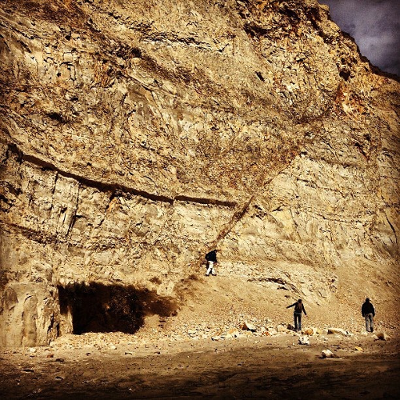# Add Second Derivative Gridding to ArcGIS Pro

299
0
07-30-2019 08:48 AM
Status: Open
Labels (1)by
New Contributor II

Other names this algorithm goes by include liner projection or projected slope. This is a surface estimation method created by Davis (1987). This is a two-part procedure, in which a weighted average of slopes projected from the nearest neighboring data points around each grid node are used to estimate the value at the node.

Initially, the slope of the surface at every data point must be estimated. The nearest n neighboring observations around a data point are found and each is weighted inversely to its distance from the data point. A linear trend surface is then fitted to these weighted observations. The constant term of the fitted regression equation is adjusted so the plane passes exactly through the data point. The slope of the trend surface is used as the local dip. If at least five points cannot be found around the data point or if the set of simultaneous equations for the fitted plane cannot be solved, the coefficients of a global linear trend are used to estimate the local slope. The slope coefficients are saved for each data point.

The second part of the algorithm estimates the value of the surface at the grid nodes. A search procedure finds n nearest neighboring data points around the node to be estimated. The X,Y coordinates of the grid node are substituted into each of the local trend surface equations associated with these data points, in effect projecting these local dipping planes to the location of the node. An average of these estimates is then calculated, weighting each slope by the inverse of the distance between the grid node and the data point associated with the slope. If a data point lies at or very near a grid intersection, the value of the data point is used directly as the value of the grid node.

This method is great for maintaining natural geologic features, such as steep slopes (generally with a dip greater than 45 deg). And, as outlined above, is an excellent method for maintaining a trend from an area with great control and projecting that trend into an area of sparse, limited, or no control.

Tags (3)